# Title 40

## SECTION 53.64

### 53.64 Test procedure: Static fractionator test.

§ 53.64 Test procedure: Static fractionator test.

(a) Overview. This test applies only to those candidate methods in which the sole deviation from the reference method is in the design of the 2.5-micron fractionation device. The purpose of this test is to ensure that the fractionation characteristics of the candidate fractionator are acceptably similar to that of the reference method sampler. It is recognized that various methodologies exist for quantifying fractionator effectiveness. The following commonly-employed techniques are provided for purposes of guidance. Other methodologies for determining sampler effectiveness may be used contingent upon prior approval by the Agency.

(1) Wash-off method. Effectiveness is determined by measuring the aerosol mass deposited on the candidate sampler's after filter versus the aerosol mass deposited in the fractionator. The material deposited in the fractionator is recovered by washing its internal surfaces. For these wash-off tests, a fluorometer must be used to quantitate the aerosol concentration. Note that if this technique is chosen, the candidate must be reloaded with coarse aerosol prior to each test point when reevaluating the curve as specified in the loading test.

(2) Static chamber method. Effectiveness is determined by measuring the aerosol mass concentration sampled by the candidate sampler's after filter versus that which exists in a static chamber. A calibrated fluorometer shall be used to quantify the collected aerosol deposits. The aerosol concentration is calculated as the measured aerosol mass divided by the sampled air volume.

(3) Divided flow method. Effectiveness is determined by comparing the aerosol concentration upstream of the candidate sampler's fractionator versus that concentration which exists downstream of the candidate fractionator. These tests may utilize either fluorometry or a real-time aerosol measuring device to determine the aerosol concentration.

(b) Technical definition. Effectiveness under static conditions is the ratio (expressed as a percentage) of the mass concentration of particles of a given size reaching the sampler filter to the mass concentration of particles of the same size existing in the test atmosphere.

(c) Facilities and equipment required - (1) Aerosol generation. Methods for generating aerosols shall be identical to those prescribed in § 53.62(c)(2).

(2) Particle delivery system. Acceptable apparatus for delivering the generated aerosols to the candidate fractionator is dependent on the effectiveness measurement methodology and shall be defined as follows:

(i) Wash-off test apparatus. The aerosol may be delivered to the candidate fractionator through direct piping (with or without an in-line mixing chamber). Validation particle size and quality shall be conducted at a point directly upstream of the fractionator.

(ii) Static chamber test apparatus. The aerosol shall be introduced into a chamber and sufficiently mixed such that the aerosol concentration within the chamber is spatially uniform. The chamber must be of sufficient size to house at least four total filter samplers in addition to the inlet of the candidate method size fractionator. Validation of particle size and quality shall be conducted on representative aerosol samples extracted from the chamber.

(iii) Divided flow test apparatus. The apparatus shall allow the aerosol concentration to be measured upstream and downstream of the fractionator. The aerosol shall be delivered to a manifold with two symmetrical branching legs. One of the legs, referred to as the bypass leg, shall allow the challenge aerosol to pass unfractionated to the detector. The other leg shall accommodate the fractionation device.

(3) Particle concentration measurement - (i) Fluorometry. Refer to § 53.62(c)(7).

(ii) Number concentration measurement. A number counting particle sizer may be used in conjunction with the divided flow test apparatus in lieu of fluorometric measurement. This device must have a minimum range of 1 to 10 µm, a resolution of 0.1 µm, and an accuracy of 0.15 µm such that primary particles may be distinguished from multiplets for all test aerosols. The measurement of number concentration shall be accomplished by integrating the primary particle peak.

(d) Setup - (1) Remove the inlet and downtube from the candidate fractionator. All tests procedures shall be conducted with the inlet and downtube removed from the candidate sampler.

(2) Surface treatment of the fractionator. Rinsing aluminum surfaces with alkaline solutions has been found to adversely affect subsequent fluorometric quantitation of aerosol mass deposits. If wash-off tests are to be used for quantifying aerosol penetration, internal surfaces of the fractionator must first be plated with electroless nickel. Specifications for this plating are specified in Society of Automotive Engineers Aerospace Material Specification (SAE AMS) 2404C, Electroless Nickel Plating (Reference 3 in appendix A of subpart F).

(e) Test procedure: Wash-off method - (1) Clean the candidate sampler. Note: The procedures in this step may be omitted if this test is being used to evaluate the fractionator after being loaded as specified in § 53.65.

(i) Clean and dry the internal surfaces of the candidate sampler.

(ii) Prepare the internal fractionator surfaces in strict accordance with the operating instructions specified in the sampler's operating manual referred to in section 7.4.18 of 40 CFR part 50, appendix L.

(2) Generate aerosol. Follow the procedures for aerosol generation prescribed in § 53.62(d)(2).

(3) Verify the quality of the test aerosol. Follow the procedures for verification of test aerosol size and quality prescribed in § 53.62(d)(4).

(4) Determine effectiveness for the particle size being produced. (i) Collect particles downstream of the fractionator on an appropriate filter over a time period such that the relative error of the fluorometric measurement is less than 5.0 percent.

(ii) Determine the quantity of material collected on the after filter of the candidate method using a calibrated fluorometer. Calculate and record the aerosol mass concentration for the sampler filter as:

Equation 20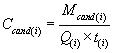where: i = replicate number; Mcand = mass of material collected with the candidate sampler; Q = candidate sampler volumetric flowrate; and t = sampling time.

(iii) Wash all interior surfaces upstream of the filter and determine the quantity of material collected using a calibrated fluorometer. Calculate and record the fluorometric mass concentration of the sampler wash as:

Equation 21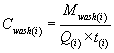where: i = replicate number; Mwash = mass of material washed from the interior surfaces of the fractionator; Q = candidate sampler volumetric flowrate; and t = sampling time.

(iv) Calculate and record the sampling effectiveness of the test sampler for this particle size as:

Equation 22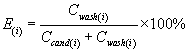where: i = replicate number.

(v) Repeat steps in paragraphs (e)(4) of this section, as appropriate, to obtain a minimum of three replicate measurements of sampling effectiveness. Note: The procedures for loading the candidate in § 53.65 must be repeated between repetitions if this test is being used to evaluate the fractionator after being loaded as specified in § 53.65.

(vi) Calculate and record the average sampling effectiveness of the test sampler as:

Equation 23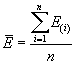where: i = replicate number; and n = number of replicates.

(vii)(A) Calculate and record the coefficient of variation for the replicate sampling effectiveness measurements of the test sampler as:

Equation 24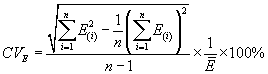where: i = replicate number; and n = total number of measurements.

(B) If the value of CVE exceeds 10 percent, then steps in paragraphs (e) (2) through (e)(4) of this section must be repeated.

(5) Repeat steps in paragraphs (e) (1) through (e)(4) of this section for each particle size specified in table F-2 of this subpart.

(f) Test procedure: Static chamber method - (1) Generate aerosol. Follow the procedures for aerosol generation prescribed in § 53.62(d)(2).

(2) Verify the quality of the test aerosol. Follow the procedures for verification of test aerosol size and quality prescribed in § 53.62(d)(4).

(3) Introduce particles into chamber. Introduce the particles into the static chamber and allow the particle concentration to stabilize.

(4) Install and operate the candidate sampler's fractionator and its after-filter and at least four total filters. (i) Install the fractionator and an array of four or more equally spaced total filter samplers such that the total filters surround and are in the same plane as the inlet of the fractionator.

(ii) Simultaneously collect particles onto appropriate filters with the total filter samplers and the fractionator for a time period such that the relative error of the measured concentration is less than 5.0 percent.

(5) Calculate the aerosol spatial uniformity in the chamber. (i) Determine the quantity of material collected with each total filter sampler in the array using a calibrated fluorometer. Calculate and record the mass concentration for each total filter sampler as:

Equation 25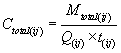where: i = replicate number; j = total filter sampler number; Mtotal = mass of material collected with the total filter sampler; Q = total filter sampler volumetric flowrate; and t = sample time.

(ii) Calculate and record the mean mass concentration as:

Equation 26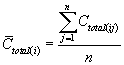where: n = total number of samplers; i = replicate number; and j = filter sampler number.

(iii) (A) Calculate and record the coefficient of variation of the total mass concentration as:

Equation 27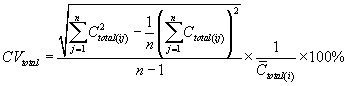where: i = replicate number; j = total filter sampler number; and n = number of total filter samplers.

(B) If the value of CVtotal exceeds 10 percent, then the particle concentration uniformity is unacceptable, alterations to the static chamber test apparatus must be made, and steps in paragraphs (f)(1) through (f)(5) of this section must be repeated.

(6) Determine the effectiveness of the candidate sampler. (i) Determine the quantity of material collected on the candidate sampler's after filter using a calibrated fluorometer. Calculate and record the mass concentration for the candidate sampler as:

Equation 28where: i = replicate number; Mcand = mass of material collected with the candidate sampler; Q = candidate sampler volumetric flowrate; and t = sample time.

(ii) Calculate and record the sampling effectiveness of the candidate sampler as:

Equation 29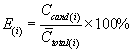where: i = replicate number.

(iii) Repeat step in paragraph (f)(4) through (f)(6) of this section, as appropriate, to obtain a minimum of three replicate measurements of sampling effectiveness.

(iv) Calculate and record the average sampling effectiveness of the test sampler as:

Equation 30where: i= replicate number.

(v)(A) Calculate and record the coefficient of variation for the replicate sampling effectiveness measurements of the test sampler as:

Equation 31where: i = replicate number; and n = number of measurements of effectiveness.

(B) If the value of CVE exceeds 10 percent, then the test run (steps in paragraphs (f)(2) through (f)(6) of this section) is unacceptable and must be repeated.

(7) Repeat steps in paragraphs (f)(1) through (f)(6) of this section for each particle size specified in table F-2 of this subpart.

(g) Test procedure: Divided flow method - (1) Generate calibration aerosol. Follow the procedures for aerosol generation prescribed in § 53.62(d)(2).

(2) Verify the quality of the calibration aerosol. Follow the procedures for verification of calibration aerosol size and quality prescribed in § 53.62(d)(4).

(3) Introduce aerosol. Introduce the calibration aerosol into the static chamber and allow the particle concentration to stabilize.

(4) Validate that transport is equal for the divided flow option. (i) With fluorometry as a detector:

(A) Install a total filter on each leg of the divided flow apparatus.

(B) Collect particles simultaneously through both legs at 16.7 L/min onto an appropriate filter for a time period such that the relative error of the measured concentration is less than 5.0 percent.

(C) Determine the quantity of material collected on each filter using a calibrated fluorometer. Calculate and record the mass concentration measured in each leg as:

Equation 32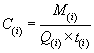where: i = replicate number, M = mass of material collected with the total filter; and Q = candidate sampler volumetric flowrate.

(D) Repeat steps in paragraphs (g)(4)(i)(A) through (g)(4)(i)(C) of this section until a minimum of three replicate measurements are performed.

(ii) With an aerosol number counting device as a detector:

(A) Remove all flow obstructions from the flow paths of the two legs.

(B) Quantify the aerosol concentration of the primary particles in each leg of the apparatus.

(C) Repeat steps in paragraphs (g)(4)(ii)(A) through (g)(4)(ii)(B) of this section until a minimum of three replicate measurements are performed.

(iii) (A) Calculate the mean concentration and coefficient of variation as:

Equation 33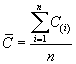Equation 34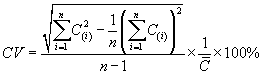where: i = replicate number; and n = number of replicates.

(B) If the measured mean concentrations through the two legs do not agree within 5 percent, then adjustments may be made in the setup, and this step must be repeated.

(5) Determine effectiveness. Determine the sampling effectiveness of the test sampler with the inlet removed by one of the following procedures:

(i) With fluorometry as a detector:

(A) Prepare the divided flow apparatus for particle collection. Install a total filter into the bypass leg of the divided flow apparatus. Install the particle size fractionator with a total filter placed immediately downstream of it into the other leg.

(B) Collect particles simultaneously through both legs at 16.7 L/min onto appropriate filters for a time period such that the relative error of the measured concentration is less than 5.0 percent.

(C) Determine the quantity of material collected on each filter using a calibrated fluorometer. Calculate and record the mass concentration measured by the total filter and that measured after penetrating through the candidate fractionator as follows:

Equation 35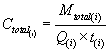Equation 36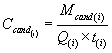where: i = replicate number.

(ii) With a number counting device as a detector:

(A) Install the particle size fractionator into one of the legs of the divided flow apparatus.

(B) Quantify and record the aerosol number concentration of the primary particles passing through the fractionator as Ccand(i).

(C) Divert the flow from the leg containing the candidate fractionator to the bypass leg. Allow sufficient time for the aerosol concentration to stabilize.

(D) Quantify and record the aerosol number concentration of the primary particles passing through the bypass leg as Ctotal(i).

(iii) Calculate and record sampling effectiveness of the candidate sampler as:

Equation 37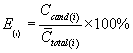where: i = replicate number.

(6) Repeat step in paragraph (g)(5) of this section, as appropriate, to obtain a minimum of three replicate measurements of sampling effectiveness.

(7) Calculate the mean and coefficient of variation for replicate measurements of effectiveness. (i) Calculate and record the mean sampling effectiveness of the candidate sampler as:

Equation 38where: i = replicate number.

(ii)(A) Calculate and record the coefficient of variation for the replicate sampling effectiveness measurements of the candidate sampler as:

Equation 39where: i = replicate number; and n = number of replicates.

(B) If the coefficient of variation is not less than 10 percent, then the test run must be repeated (steps in paragraphs (g)(1) through (g)(7) of this section).

(8) Repeat steps in paragraphs (g)(1) through (g)(7) of this section for each particle size specified in table F-2 of this subpart.

(h) Calculations - (1) Treatment of multiplets. For all measurements made by fluorometric analysis, data shall be corrected for the presence of multiplets as described in § 53.62(f)(1). Data collected using a real-time device (as described in paragraph (c)(3)(ii)) of this section will not require multiplet correction.

(2) Cutpoint determination. For each wind speed determine the sampler Dp50 cutpoint defined as the aerodynamic particle size corresponding to 50 percent effectiveness from the multiplet corrected smooth curve.

(3) Graphical analysis and numerical integration with ambient distributions. Follow the steps outlined in § 53.62 (e)(3) through (e)(4) to calculate the estimated concentration measurement ratio between the candidate sampler and a reference method sampler.

(i) Test evaluation. The candidate method passes the static fractionator test if the values of Rc and Dp50 for each distribution meets the specifications in table F-1 of this subpart.

[62 FR 38814, July 18, 1997; 63 FR 7714, Feb. 17, 1998]